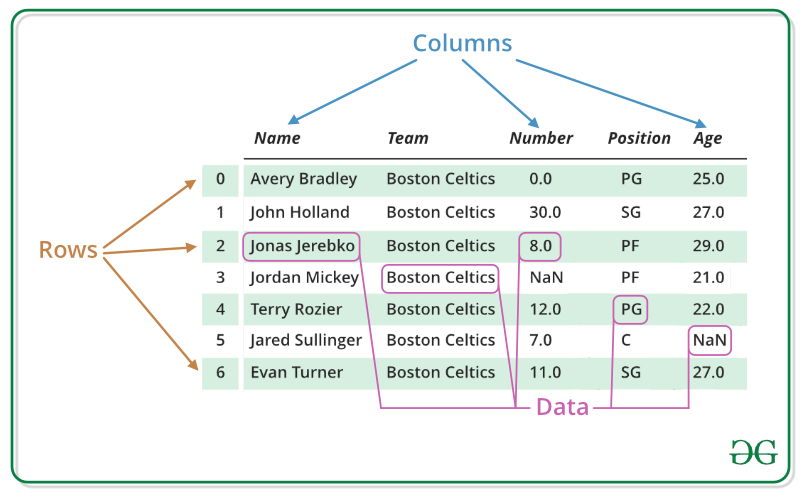# R – Data Frames

R is an open-source programming language that is widely used as a statistical software and data analysis tool. DataFrames in R are generic data objects of R which are used to store the tabular data. Data frames can also be interpreted as mattresses where each column of a matrix can be of the different data types. DataFrame is made up of three principal components, the data, rows, and columns.#### Create Dataframe

To create a data frame in R use `data.frame()` command and then pass each of the vectors you have created as arguments to the function.
Example:

 `# R program to create dataframe ` ` `  `# creating a data frame ` `friend.data <``-` `data.frame( ` `    ``friend_id ``=` `c(``1``:``5``),  ` `    ``friend_name ``=` `c(``"Sachin"``, ``"Sourav"``,  ` `                    ``"Dravid"``, ``"Sehwag"``,  ` `                    ``"Dhoni"``), ` `    ``stringsAsFactors ``=` `FALSE ` `) ` `# print the data frame ` `print``(friend.data) `

Output:

```      friend_id friend_name
1         1      Sachin
2         2      Sourav
3         3      Dravid
4         4      Sehwag
5         5       Dhoni
```

#### Get the Structure of the Data Frame

One can get the structure of the data frame using `str()` function in R. It can display even the internal structure of large lists which are nested. It provides one liner output for the basic R objects letting the user know about the object and its constituents.
Example:

 `# R program to get the ` `# structure of the data frame ` ` `  `# creating a data frame ` `friend.data <``-` `data.frame( ` `    ``friend_id ``=` `c(``1``:``5``),  ` `    ``friend_name ``=` `c(``"Sachin"``, ``"Sourav"``,  ` `                    ``"Dravid"``, ``"Sehwag"``,  ` `                    ``"Dhoni"``), ` `    ``stringsAsFactors ``=` `FALSE ` `) ` `# using str() ` `print``(``str``(friend.data)) `

Output:

```'data.frame':    5 obs. of  2 variables:
\$ friend_id  : int  1 2 3 4 5
\$ friend_name: chr  "Sachin" "Sourav" "Dravid" "Sehwag" ...
NULL
```

#### Summary of data in the data frame

In R data frame, the statistical summary and nature of the data can be obtained by applying `summary()` function. It is a generic function used to produce result summaries of the results of various model fitting functions. The function invokes particular methods which depend on the class of the first argument.
Example:

 `# R program to get the ` `# summary of the data frame ` ` `  `# creating a data frame ` `friend.data <``-` `data.frame( ` `    ``friend_id ``=` `c(``1``:``5``),  ` `    ``friend_name ``=` `c(``"Sachin"``, ``"Sourav"``,  ` `                    ``"Dravid"``, ``"Sehwag"``,  ` `                    ``"Dhoni"``), ` `    ``stringsAsFactors ``=` `FALSE ` `) ` `# using summary() ` `print``(summary(friend.data))  `

Output:

```    friend_id friend_name
Min.   :1   Length:5
1st Qu.:2   Class :character
Median :3   Mode  :character
Mean   :3
3rd Qu.:4
Max.   :5
```

#### Extract Data from Data Frame

Extract data from a data frame means that to access its rows or columns. One can extract specific column from a data frame using it’s column name.
Example:

 `# R program to extract ` `# data from the data frame ` ` `  `# creating a data frame ` `friend.data <``-` `data.frame( ` `    ``friend_id ``=` `c(``1``:``5``),  ` `    ``friend_name ``=` `c(``"Sachin"``, ``"Sourav"``,  ` `                    ``"Dravid"``, ``"Sehwag"``,  ` `                    ``"Dhoni"``), ` `    ``stringsAsFactors ``=` `FALSE ` `) ` ` `  `# Extracting friend_name column ` `result <``-` `data.frame(friend.data\$friend_name) ` `print``(result) `

Output:

```    friend.data.friend_name
1                  Sachin
2                  Sourav
3                  Dravid
4                  Sehwag
5                   Dhoni
```

#### Expand Data Frame

A data frame in R can be expanded by adding new columns and rows to the already existing data frame.
Example:

 `# R program to expand ` `# the data frame ` ` `  `# creating a data frame ` `friend.data <``-` `data.frame( ` `    ``friend_id ``=` `c(``1``:``5``),  ` `    ``friend_name ``=` `c(``"Sachin"``, ``"Sourav"``,  ` `                    ``"Dravid"``, ``"Sehwag"``,  ` `                    ``"Dhoni"``), ` `    ``stringsAsFactors ``=` `FALSE ` `) ` ` `  `# Expanding data frame ` `friend.data\$location <``-` `c(``"Kolkata"``, ``"Delhi"``,  ` `                       ``"Bangalore"``, ``"Hyderabad"``, ` `                       ``"Chennai"``) ` `resultant <``-` `friend.data ` `# print the modified data frame ` `print``(resultant) `

Output:

```    friend_id friend_name  location
1         1      Sachin   Kolkata
2         2      Sourav     Delhi
3         3      Dravid Bangalore
5         5       Dhoni   Chennai
```

In R, one can perform various types of operation on a data frame like accessing rows and columns, selecting the subset of the data frame, editing data frames, delete rows and columns in a data frame, etc. Please refer to DataFrame Operations in R to know about all types of operations that can be performed on a data frame.

My Personal Notes arrow_drop_upCheck out this Author's contributed articles.

If you like GeeksforGeeks and would like to contribute, you can also write an article using contribute.geeksforgeeks.org or mail your article to contribute@geeksforgeeks.org. See your article appearing on the GeeksforGeeks main page and help other Geeks.

Please Improve this article if you find anything incorrect by clicking on the "Improve Article" button below.

Article Tags :

Be the First to upvote.

Please write to us at contribute@geeksforgeeks.org to report any issue with the above content.